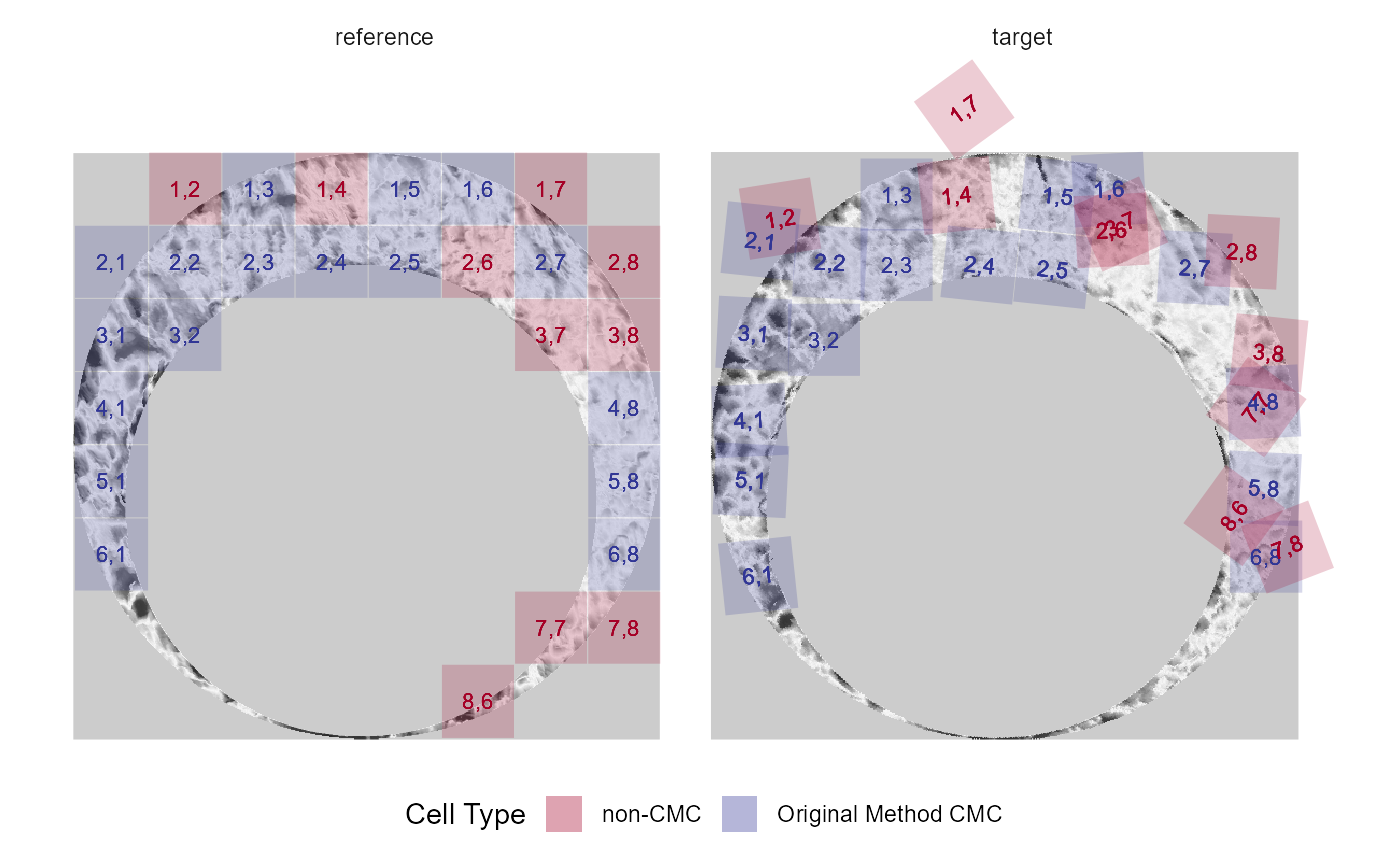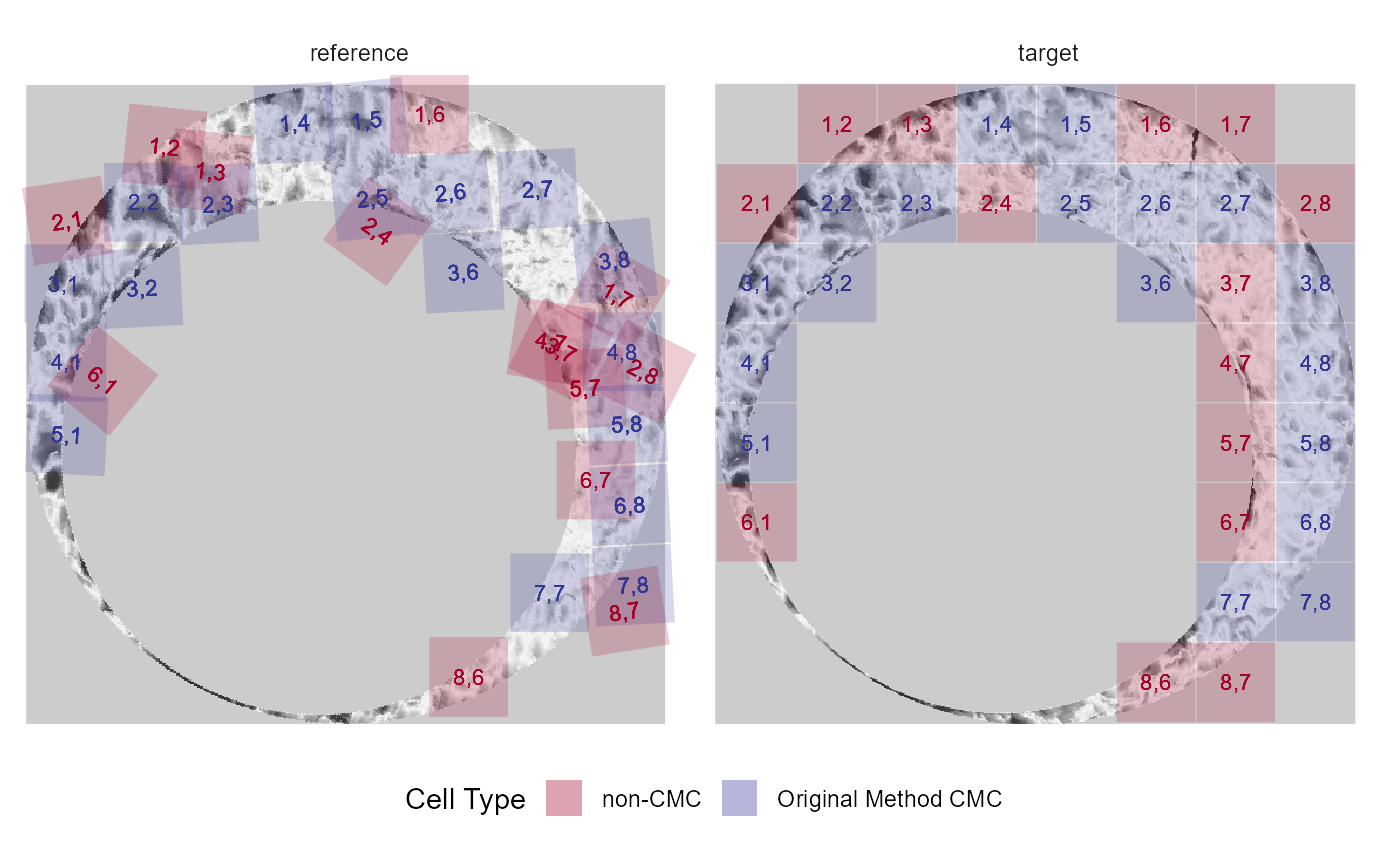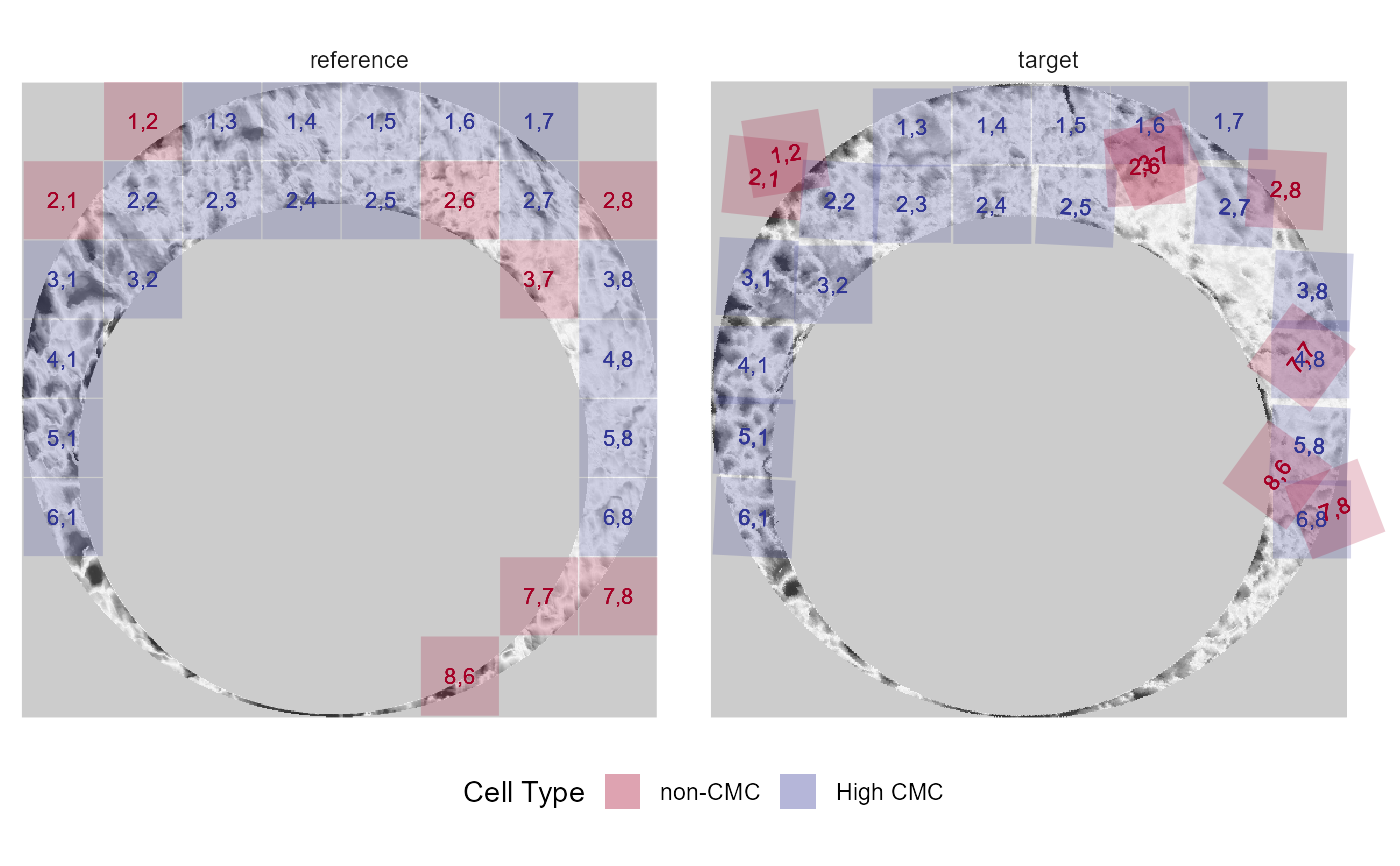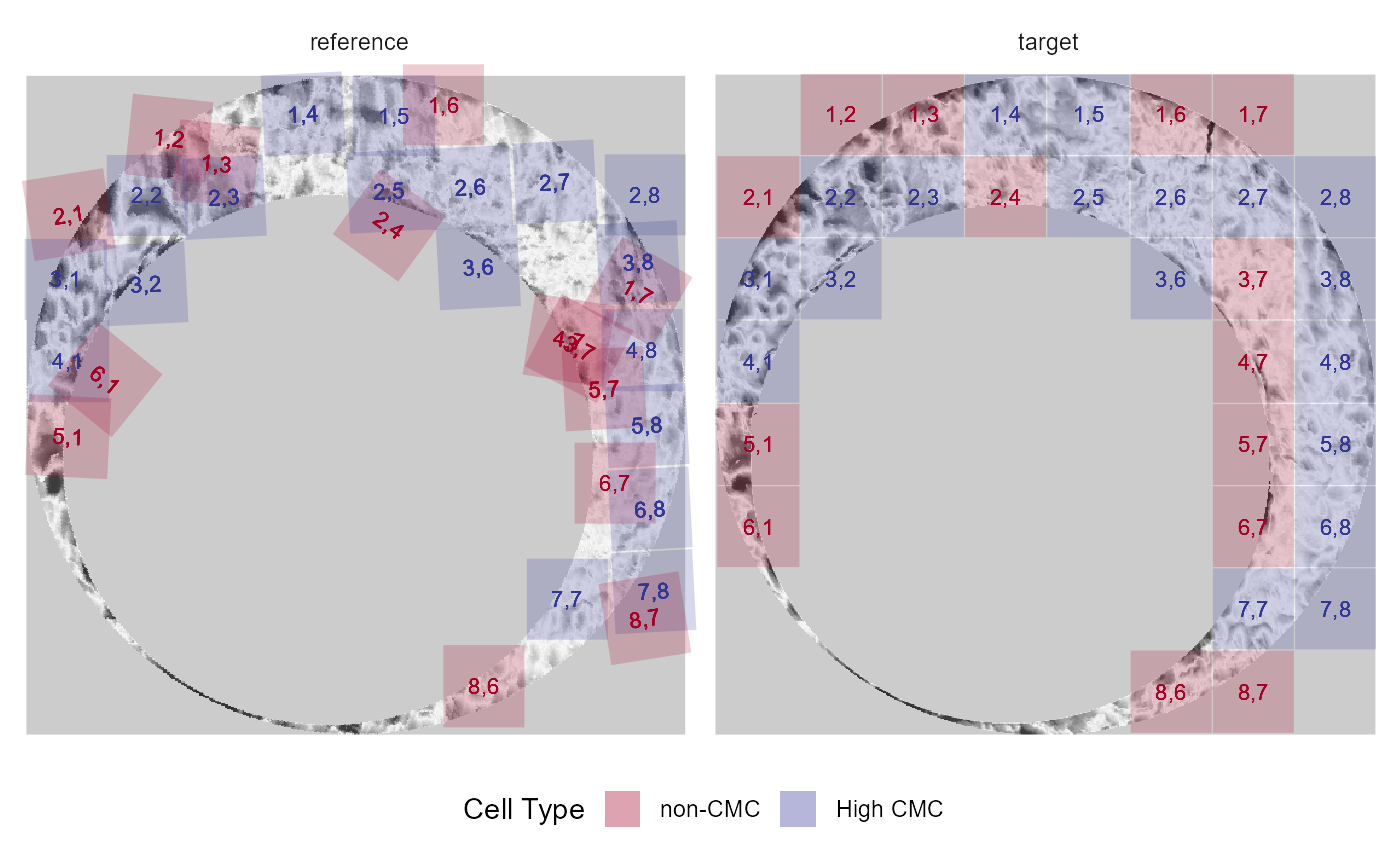Constructs either a single faceted plot or a list of plots depicting the CMCs/non-CMCs under the initially proposed and High CMC methods for a pair of cartridge case scans

cmcPlot(
reference,
target,
reference_v_target_CMCs,
target_v_reference_CMCs = reference_v_target_CMCs,
corColName = "pairwiseCompCor",
type = "faceted",
x3pNames = c("reference", "target"),
legend.quantiles = c(0, 0.01, 0.25, 0.5, 0.75, 0.99, 1),
height.colors = c("#1B1B1B", "#404040", "#7B7B7B", "#B0B0B0", "#DBDBDB", "#F7F7F7",
"#E4E4E4", "#C5C5C5", "#999999", "#717171", "#4E4E4E"),
cell.colors = c("#a50026", "#313695"),
cell.alpha = 0.2,
numCells = 64,
na.value = "gray80"
)

## Arguments

reference an x3p object a different x3p object CMCs for the comparison between the reference scan and the target scan. (optional) CMCs for the comparison between the target scan and the reference scan. If this is missing, then only the original method CMCs will be plotted name of correlation similarity score column used to identify the CMCs in the two comparison_*_df data frames (e.g., pairwiseCompCor) argument to be passed to cmcR::x3pListPlot function (Optional) Names of x3p objects to be included in x3pListPlot function vector of quantiles to be shown as tick marks on legend plot vector of colors to be passed to scale_fill_gradientn that dictates the height value colorscale vector of 2 colors for plotting non-matching and matching (in that order) cells sets alpha of cells (passed to geom_polygon) the size of the grid used to compare the reference and target scans. Must be a perfect square. color to be used for NA values (passed to scale_fill_gradientn)

## Value

A list of 4 ggplot objects showing the CMCs identified under both decision rules and in both comparison directions.

## Examples

#Takes > 5 seconds to run
# \donttest{

comparisonDF_1to2 <- purrr::map_dfr(seq(-30,30,by = 3),
theta = .))
comparisonDF_2to1 <- purrr::map_dfr(seq(-30,30,by = 3),
theta = .))

comparisonDF_1to2 <- comparisonDF_1to2 %>%
dplyr::mutate(originalMethodClassif = decision_CMC(cellIndex = cellIndex,
x = x,
y = y,
theta = theta,
corr = pairwiseCompCor),
highCMCClassif = decision_CMC(cellIndex = cellIndex,
x = x,
y = y,
theta = theta,
corr = pairwiseCompCor,
tau = 1))

comparisonDF_2to1 <- comparisonDF_2to1 %>%
dplyr::mutate(originalMethodClassif = decision_CMC(cellIndex = cellIndex,
x = x,
y = y,
theta = theta,
corr = pairwiseCompCor),
highCMCClassif = decision_CMC(cellIndex = cellIndex,
x = x,
y = y,
theta = theta,
corr = pairwiseCompCor,
tau = 1))

#> $originalMethodCMCs_reference_v_target#> #>$originalMethodCMCs_target_v_reference#>
#> $highCMC_reference_v_target#> #>$highCMC_target_v_reference#> # }